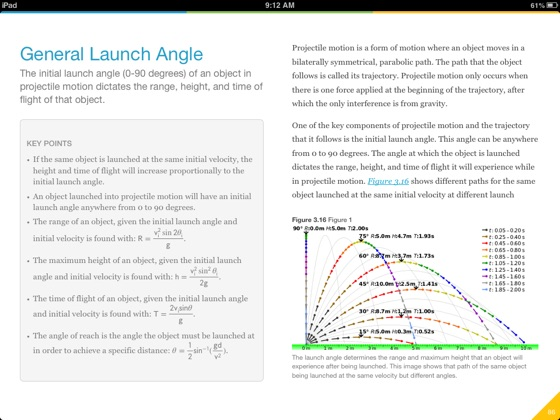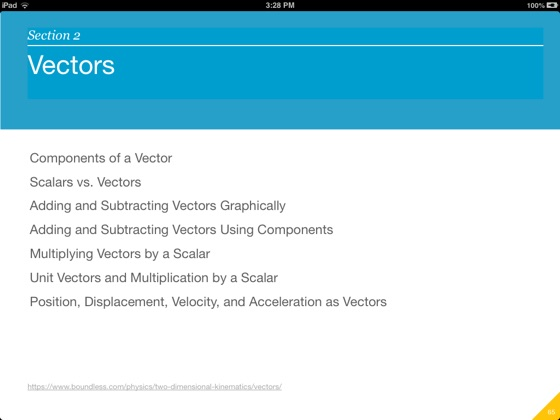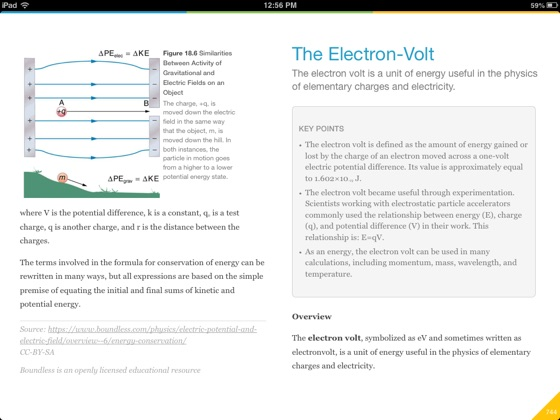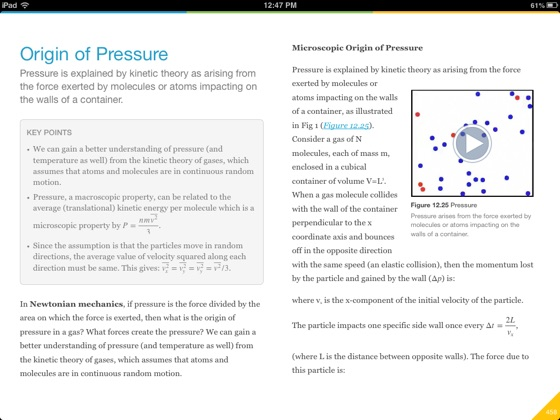• £5.99

## Publisher Description

Introduction to Physics is a college-level, introductory textbook that covers the complex and intriguing subject of Physics. Boundless works with subject matter experts to select the best open educational resources available on the web, review the content for quality, and create introductory, college-level textbooks designed to meet the study needs of university students.

This textbook covers:

Introduction -- The Basics of Physics, Units, Significant Figures and Order of Magnitude, Solving Physics Problems

Kinematics -- Basics of Kinematics, Speed and Velocity, Acceleration, Problem-Solving for Basic Kinematics, Free-Falling Objects

Two-Dimensional Kinematics -- Motion in Two Dimensions, Vectors, Projectile Motion, Multiple Velocities

The Laws of Motion -- Introduction, Force and Mass, Newton's Laws, Other Examples of Forces, Problem-Solving, Vector Nature of Forces, Further Applications of Newton's Laws

Uniform Circular Motion and Gravitation -- Introduction to UCM and Gravitation, Non-Uniform Circular Motion, Velocity, Acceleration, and Force, Types of Forces in Nature, Newton's Law of Universal Gravitation, Kepler's Laws, Gravitational Potential Energy, Energy Conservation, Angular vs. Linear Quantities

Work and Energy -- Introduction, Work Done by a Constant Force, Work Done by a Variable Force, Work-Energy Theorem, Potential Energy and Conservation of Energy, Power, CASE STUDY: World Energy Use, Further Topics

Linear Momentum and Collisions -- Introduction, Conservation of Momentum, Collisions, Rocket Propulsion, Center of Mass

Static Equilibrium, Elasticity, and Torque -- Introduction, Conditions for Equilibrium, Stability, Solving Statics Problems, Applications of Statics, Elasticity, Stress, Strain, and Fracture, The Center of Gravity, Torque and Angular Acceleration

Rotational Kinematics, Angular Momentum, and Energy -- Quantities of Rotational Kinematics, Angular Acceleration, Rotational Kinematics, Dynamics, Rotational Kinetic Energy, Conservation of Angular Momentum, Vector Nature of Rotational Kinematics, Problem Solving, Linear and Rotational Quantities, Conservation of Energy

Fluids -- Introduction, Density and Pressure, Archimedes' Principle, Cohesion and Adhesion, Fluids in Motion, Deformation of Solids

Fluid Dynamics and Its Applications -- Overview, Flow in Tubes, Bernoulli's Equation, Other Applications

Temperature and Kinetic Theory -- Introduction, Temperature and Temperature Scales, Thermal Expansion, Ideal Gas Law, Kinetic Theory, Phase Changes, The Zeroth Law of Thermodynamics, Thermal Stresses, Diffusion

Heat and Heat Transfer -- Introduction, Specific Heat, Phase Change and Latent Heat, Methods of Heat Transfer, Global Warming, Phase Equilbrium

Thermodynamics -- Introduction, The First Law of Thermodynamics, The Second Law of Thermodynamics, Entropy, The Third Law of Thermodynamics

Waves and Vibrations -- Introduction, Hooke's Law, Periodic Motion, Damped and Driven Oscillations, Waves, Wave Behavior and Interaction, Waves on Strings

Sound -- Introduction, Sound Intensity and Level, Doppler Effect and Sonic Booms, Interactions with Sound Waves, Further Topics

Electric Charge and Field -- Overview, Shelding and Charging Through Induction, Coulomb's Law, The Electric Field Revisited, Electric Flux and Gauss's Law, Applications of Electrostatics

Electric Potential and Electric Field -- Overview, Equipotential Surfaces and Lines, Point Charge, Capacitors and Dielectrics, Applications

Electric Current and Resistance -- Overview, Electric Current, Resistance and Resistors, Electric Power and Energy, Alternating Currents, Electricity in the World

Circ

••••